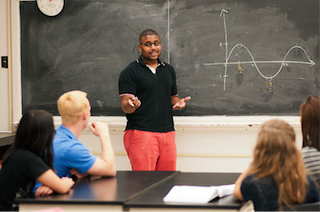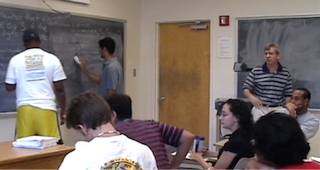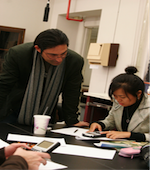## History of IBL at UT

In the late 1990's some faculty members asked the Undergraduate Studies Committee to address the perceived problem that upper division mathematics classes could not be taught at the desired level because the students failed to have the requisite mathematical maturity. A committee was formed. We decided that the basic issues were that the students were not skillful enough to prove theorems on their own, to take a proof and decide whether it is correct, and to appreciate how mathematical ideas are created and explored.

The committees response to this issue was to develop a number theory class that uses a guided discovery approach, sometimes nicknamed Inquiry Based Learning (IBL). This Modified Moore Method has students prove and write up their proofs of theorems in introductory number theory on their own and present their work to the class. The teachers job is to engage the whole class in responding to the mathematical arguments. Students in the class are asked to respond to the arguments they have just seen by pointing out gaps or errors, by restating parts of the proofs, and by determining whether the proofs are correct. The daily experiences of producing proofs and actively evaluating and understanding proofs help students to develop mathematical maturity.Introductory number theory is an ideal mathematical topic to use for teaching students to prove theorems, because it involves integers, with which they are familiar, and the early theorems isolate basic strategies such as unpacking definitions, doing a small logical step, and then using the definitions again to deduce the theorems conclusion. We have also developed IBL courses in analysis, discrete mathematics, introduction to abstract mathematics, and topology.The goal of this technique of instruction is to cause students to change from being purely consumers of mathematics to producers of mathematical concepts and proofs. IBL classes give students experience with some aspects of independent research. Many students in these classes have found the experience beneficial. A qualitative research study in the College of Education, directed by Professor Jennifer Smith, indicates that students do have beneficial changes in skill and attitude owing to the method.